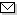Language:   Search:   ContactSearch and Browse the MSC 2000

Search:
Please note that in the second half of 2009, MSC2010 is deployed in the production of ZMATH and will become official standard in January 2010.

Enter a query and click »Search«...

90-XX Operations research, mathematical programming → ZMATH
90Cxx Mathematical programming [See also 49Mxx, 65Kxx] → ZMATH
90C05 Linear programming → ZMATH
90C06 Large-scale problems → ZMATH
90C08 Special problems of linear programming (transportation, multi-index, etc.) → ZMATH
90C09 Boolean programming → ZMATH
90C10 Integer programming → ZMATH
90C11 Mixed integer programming → ZMATH
90C15 Stochastic programming → ZMATH
90C20 Quadratic programming → ZMATH
90C22 Semidefinite programming → ZMATH
90C25 Convex programming → ZMATH
90C26 Nonconvex programming → ZMATH
90C27 Combinatorial optimization → ZMATH
90C29 Multi-objective and goal programming → ZMATH
90C30 Nonlinear programming → ZMATH
90C31 Sensitivity, stability, parametric optimization → ZMATH
90C32 Fractional programming → ZMATH
90C33 Complementarity problems → ZMATH
90C34 Semi-infinite programming → ZMATH
90C35 Programming involving graphs or networks [See also 90C27] → ZMATH
90C39 Dynamic programming [See also 49L20] → ZMATH
90C40 Markov and semi-Markov decision processes → ZMATH
90C46 Optimality conditions, duality [See also 49N15] → ZMATH
90C47 Minimax problems [See also 49K35] → ZMATH
90C48 Programming in abstract spaces → ZMATH
90C49 Extreme-point and pivoting methods → ZMATH
90C51 Interior-point methods → ZMATH
90C52 Methods of reduced gradient type → ZMATH
90C53 Methods of quasi-Newton type → ZMATH
90C55 Methods of successive quadratic programming type → ZMATH
90C56 Derivative-free methods → ZMATH
90C57 Polyhedral combinatorics, branch-and-bound, branch-and-cut → ZMATH
90C59 Approximation methods and heuristics → ZMATH
90C60 Abstract computational complexity for mathematical programming problems [See also 68Q25] → ZMATH
90C70 Fuzzy programming → ZMATH
90C90 Applications of mathematical programming → ZMATH
90C99 None of the above, but in this section → ZMATH

NewsAbel prize 2010I. M. Gelfand 1913-2009MSC2010

Copyright © 2019 Zentralblatt MATH | European Mathematical Society | FIZ Karlsruhe | Heidelberg Academy of Sciences